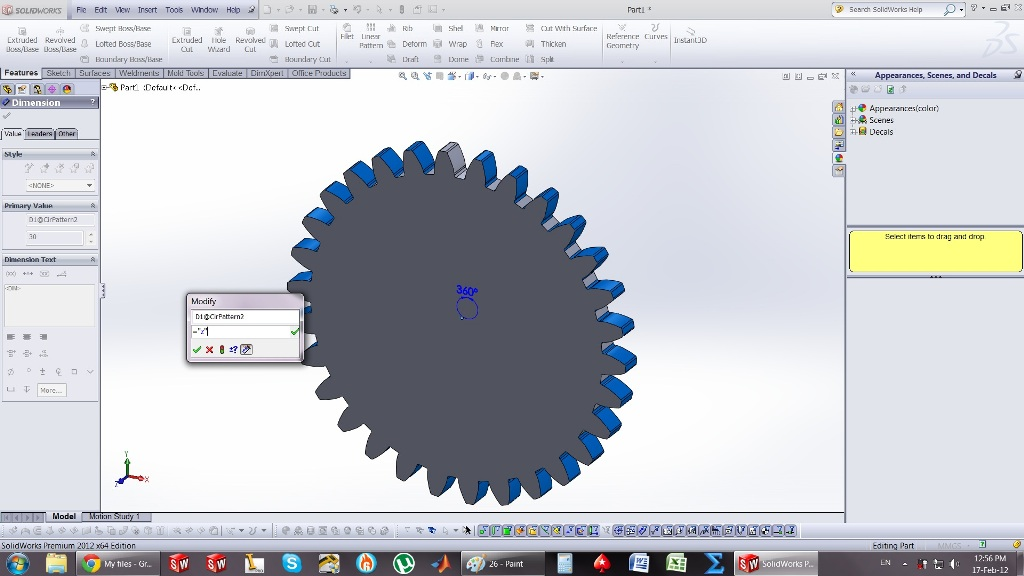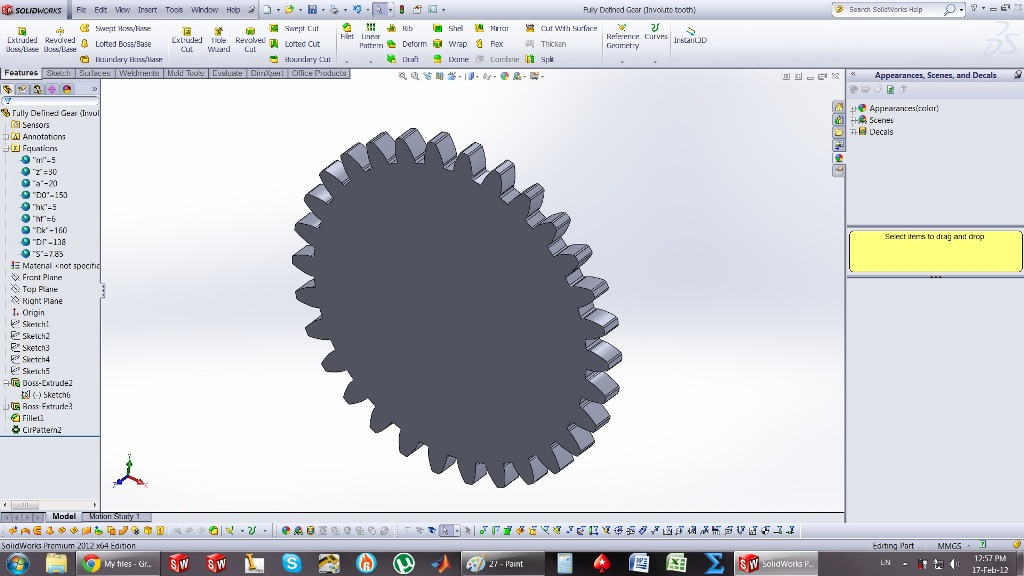# How to model Involute Gear in Solidworks?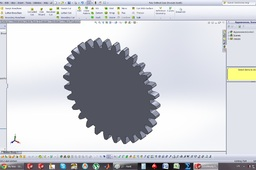these are the steps...

1. ### Step 1:

First of all we need to add some equations... so Tools -> Equations ->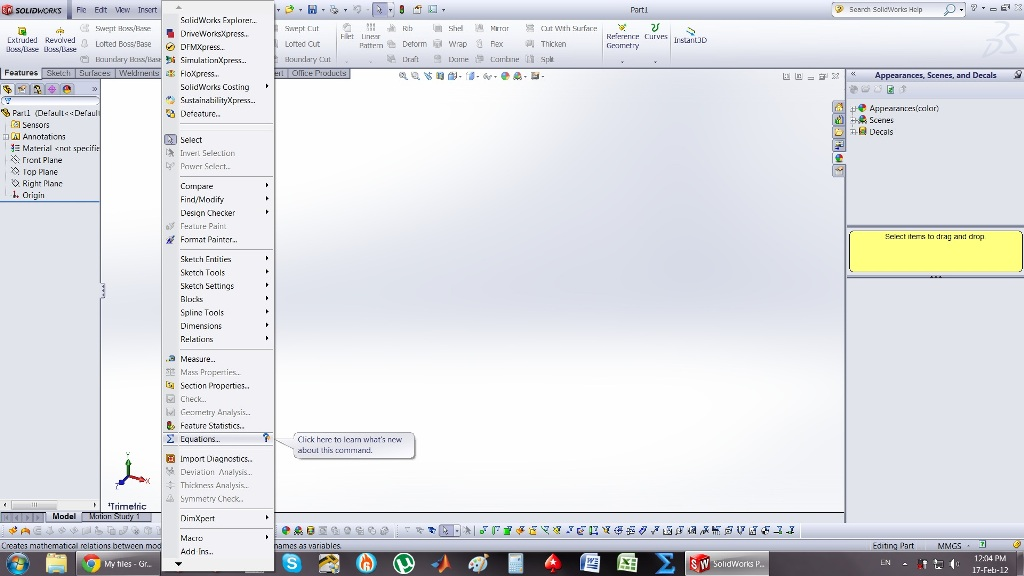2. ### Step 2:

We add the following equations... (module and No. of teeth is up to you)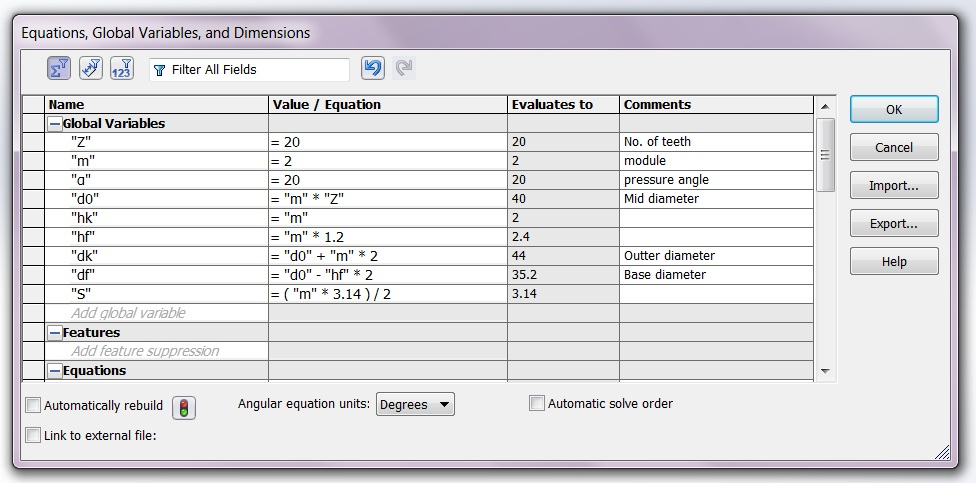3. ### Step 3:

After we add all equations, we click ok and then we start a new sketch on front plane... We create a construction line vertical...(Random length)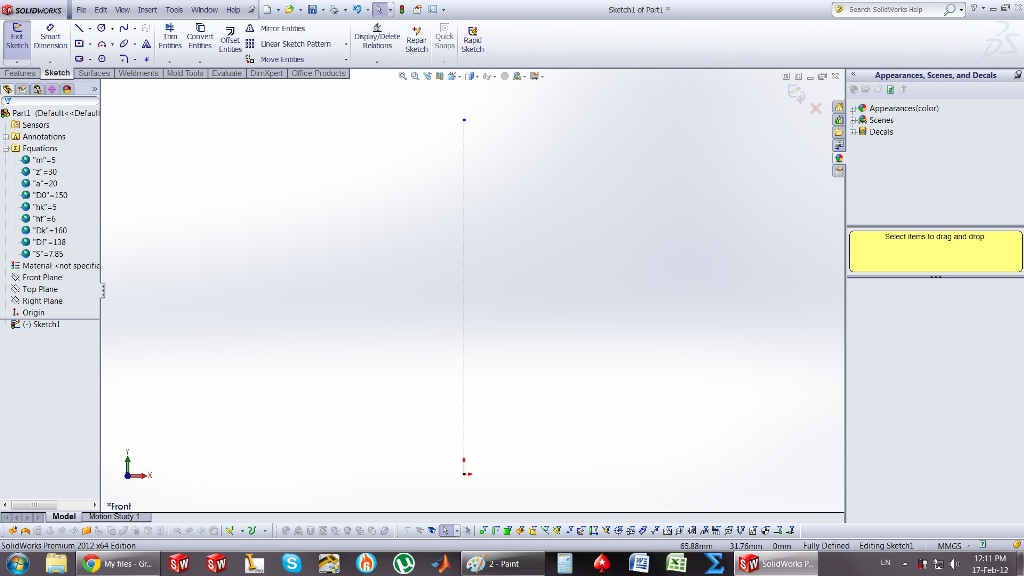4. ### Step 4:

Then we create a horizontal construction line at the length of the D0.. and then we draw a circle.. We make the circle defined by linking the dimension with the equation..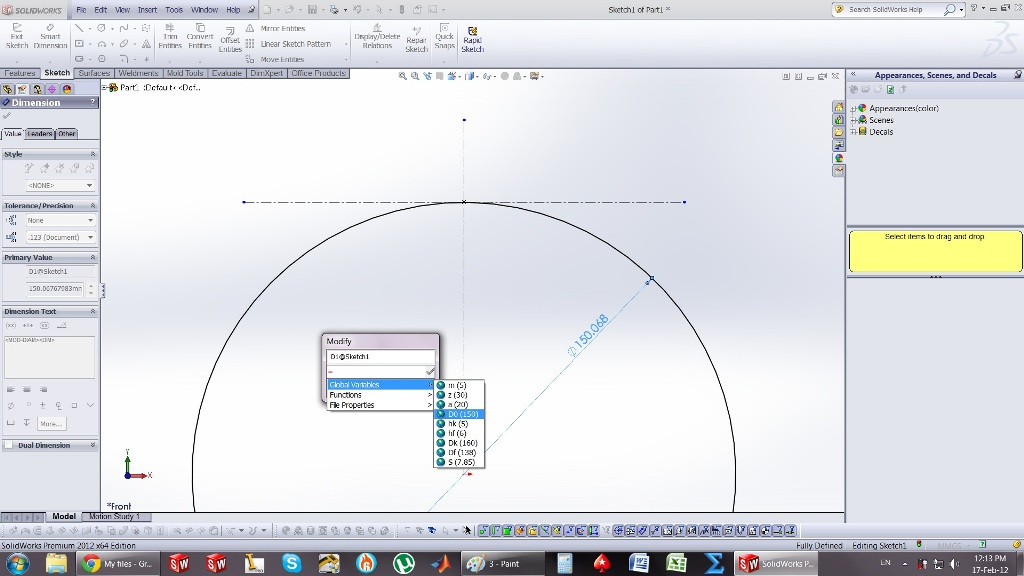5. ### Step 5:

Next we create another construction line with an angle of a(degrees)...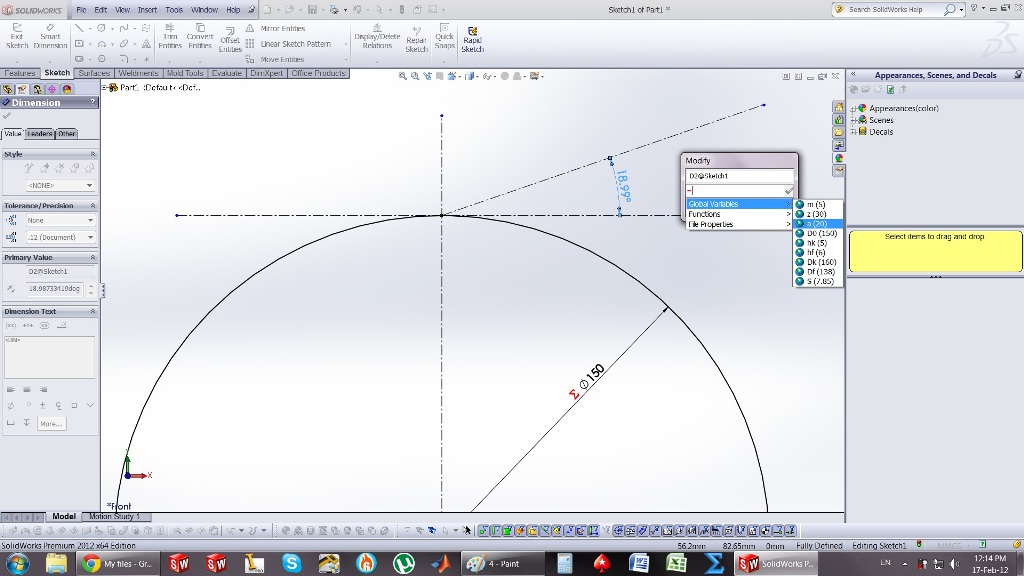6. ### Step 6:

We create another one on the other side and make them co-linear. Then we draw another one from the cinter of the circle and make it perpendicular...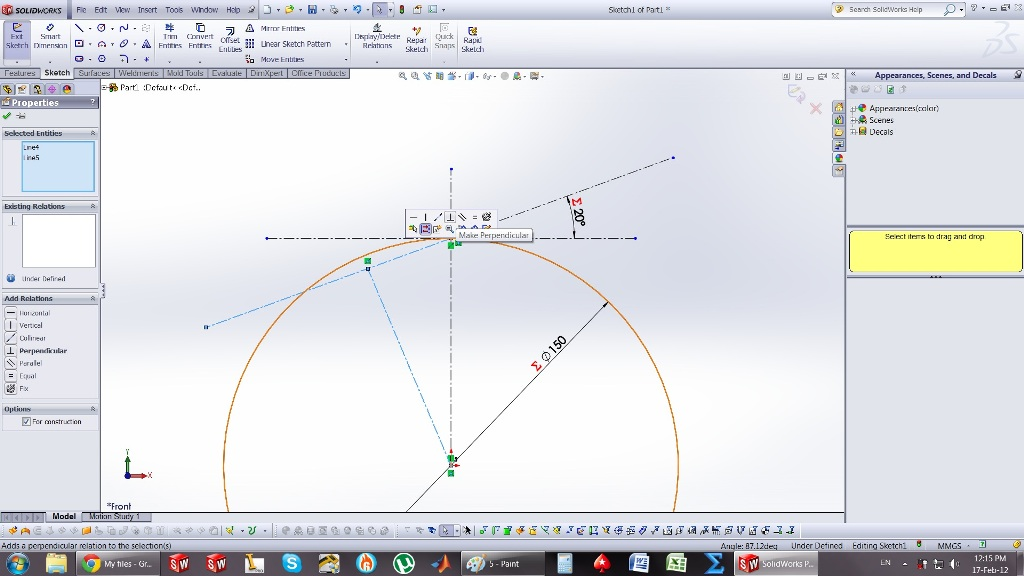7. ### Step 7:

We draw a circle from the center to that point. We then draw 5 construction lines from the center of the circle dividing the arc into 5 pieces...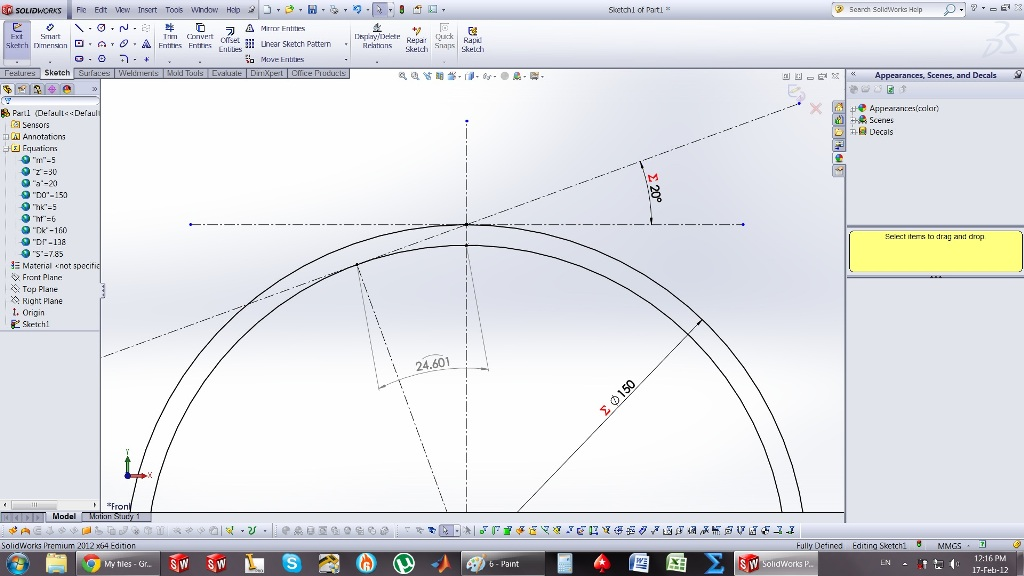8. ### Step 8:

After, we divide the line above into 5 pieces with points...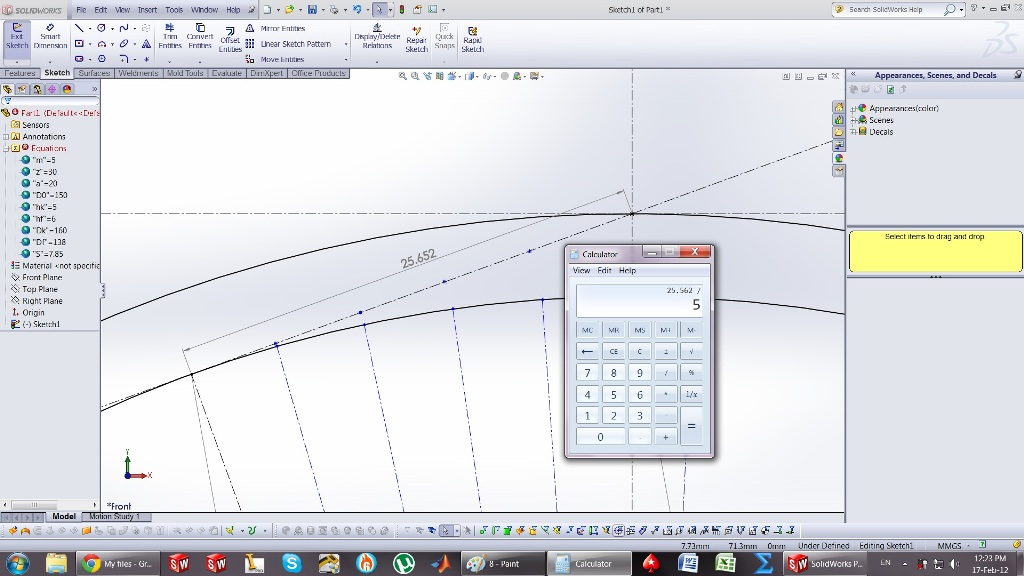9. ### Step 9:

And we make the sections equal.. after that, we link the section dimensions, with the arc sections below...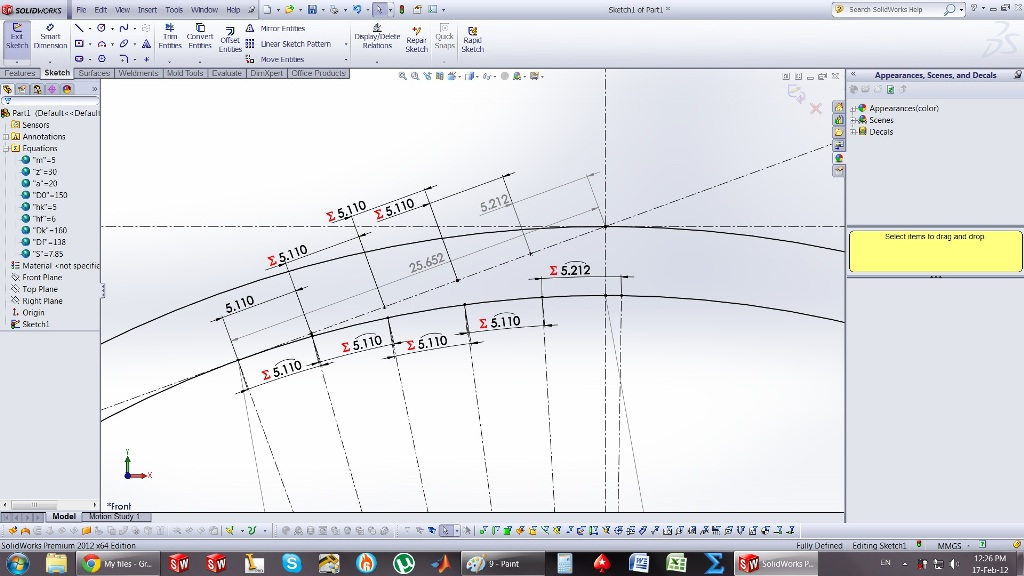10. ### Step 10:

Next we draw construction lines, from every point of the arcs, and make them tangent to the circle... dimension them as in the picture...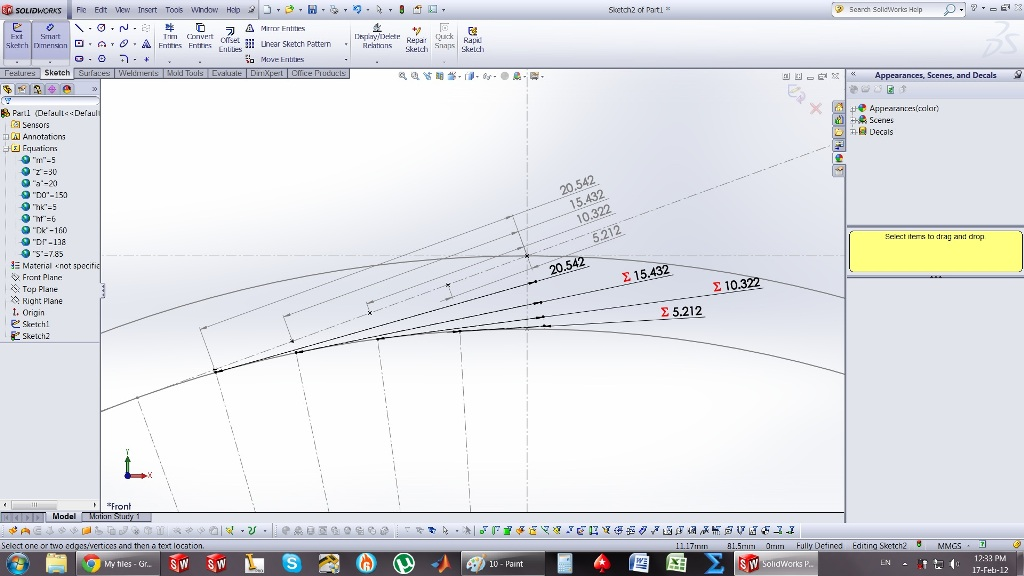11. ### Step 11:

Same thing for the back side...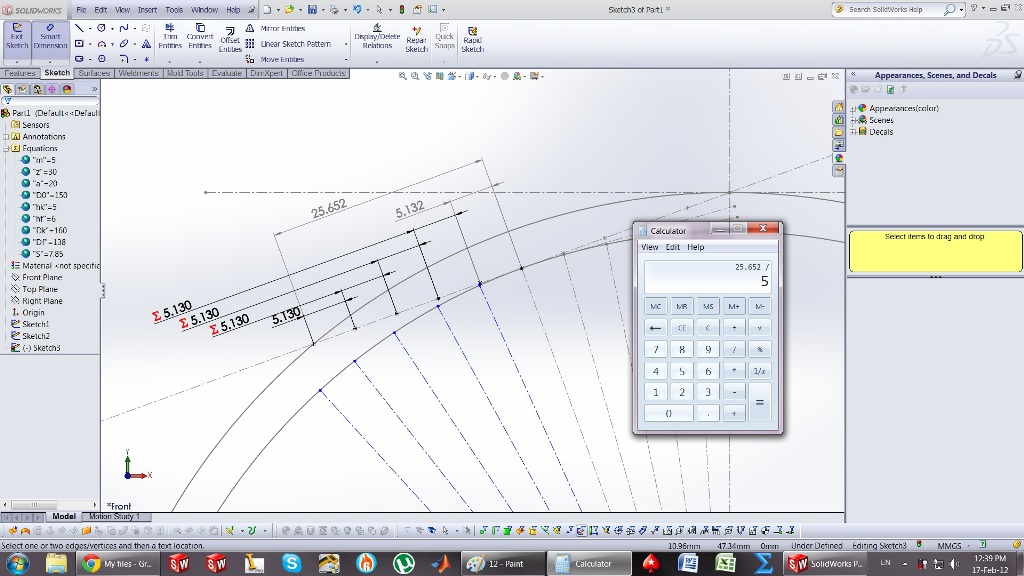12. ### Step 12:

spliting...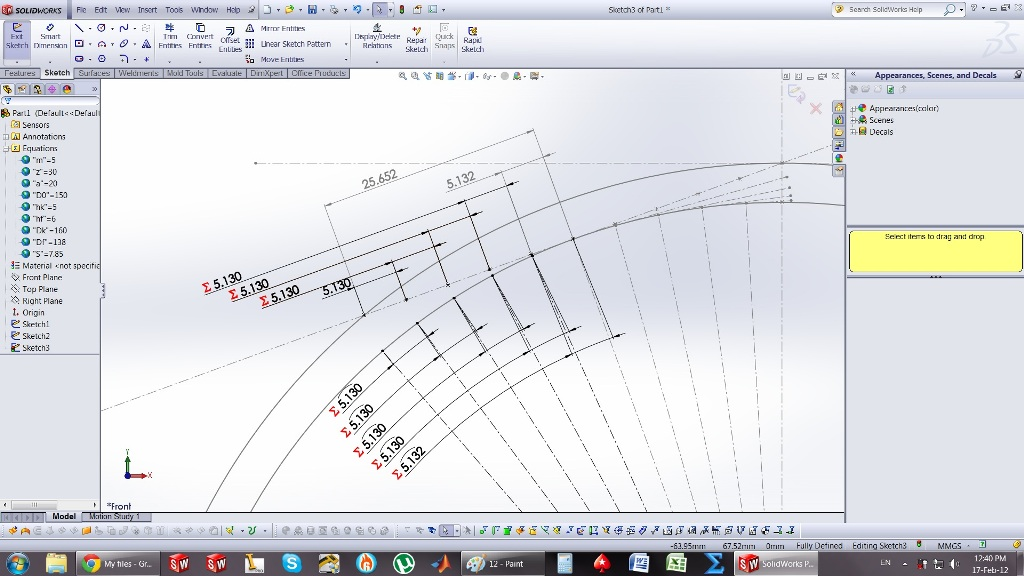13. ### Step 13:

tangent lines...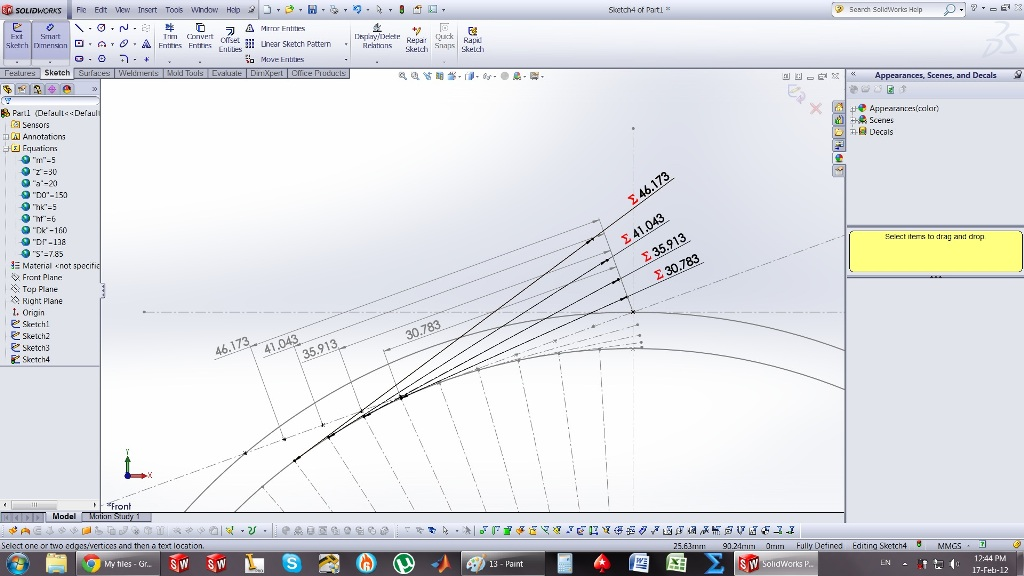14. ### Step 14:

next we create top circle of the gear... and link the dimension to the equation shown in the picture...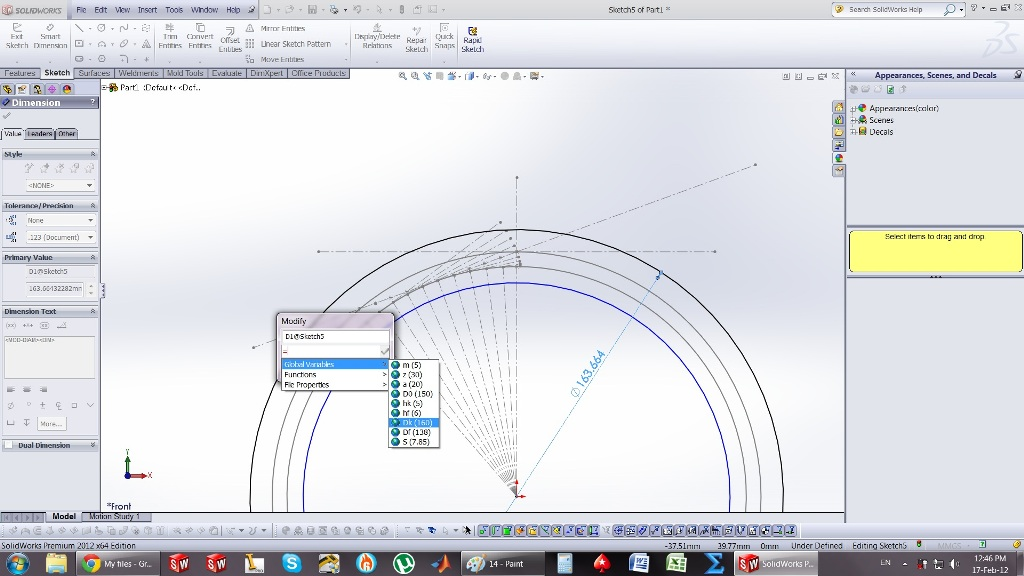15. ### Step 15:

same at here...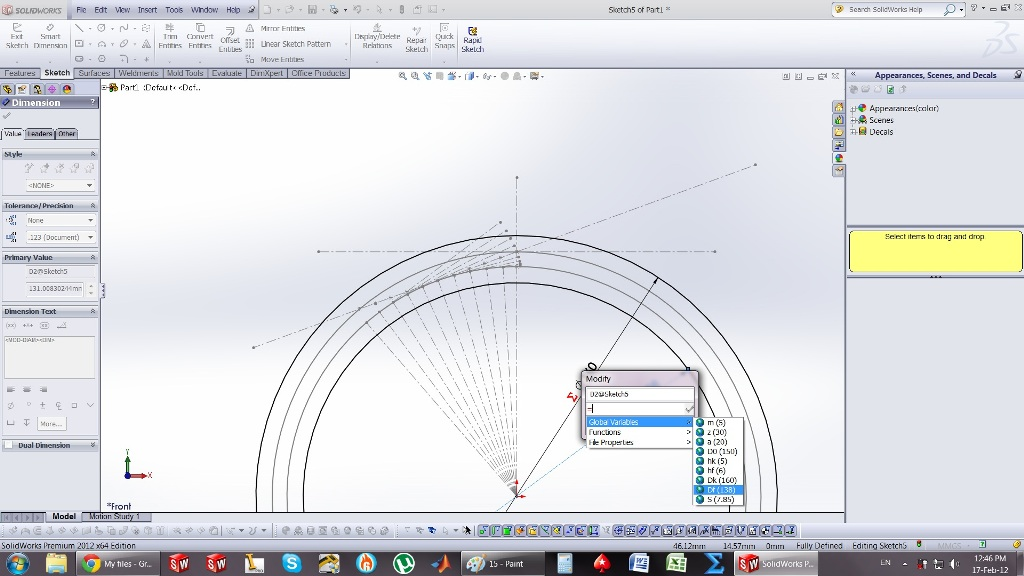16. ### Step 16:

select spline, and join the end points of the contsruction lines...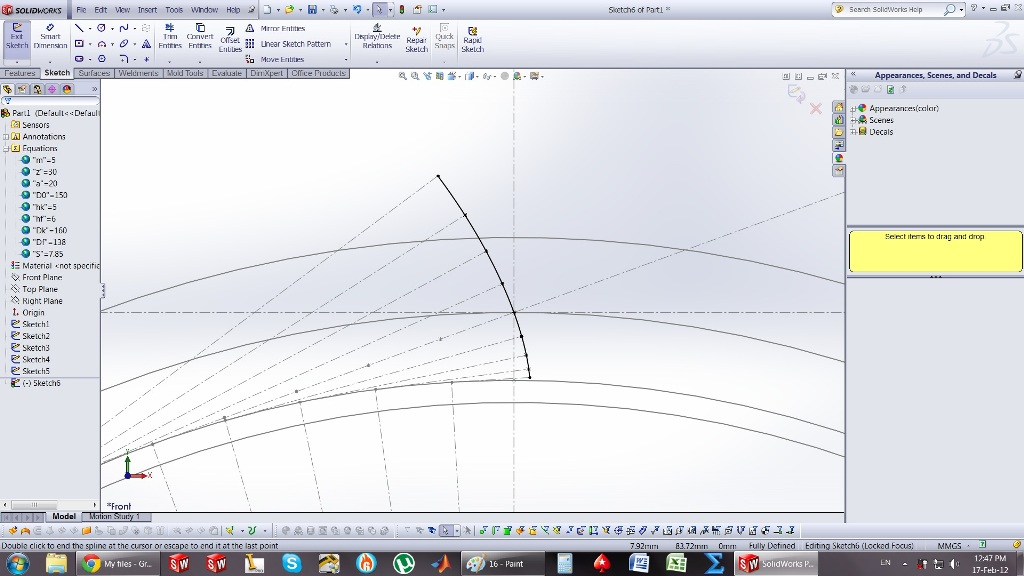17. ### Step 17:

Join the lower end of the spline with the center of the circle with a line..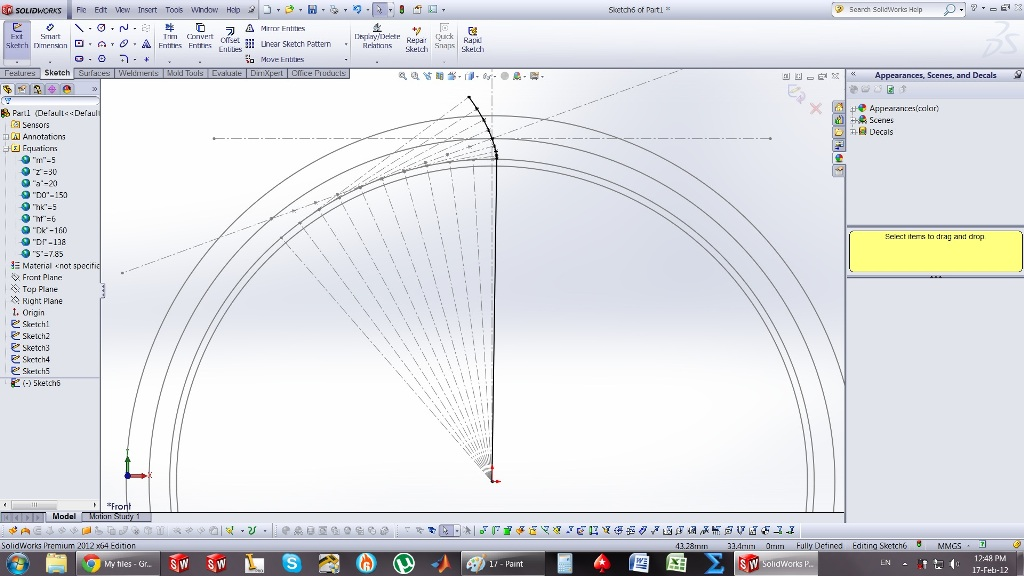18. ### Step 18:

create a construction line in the point shown in the picture..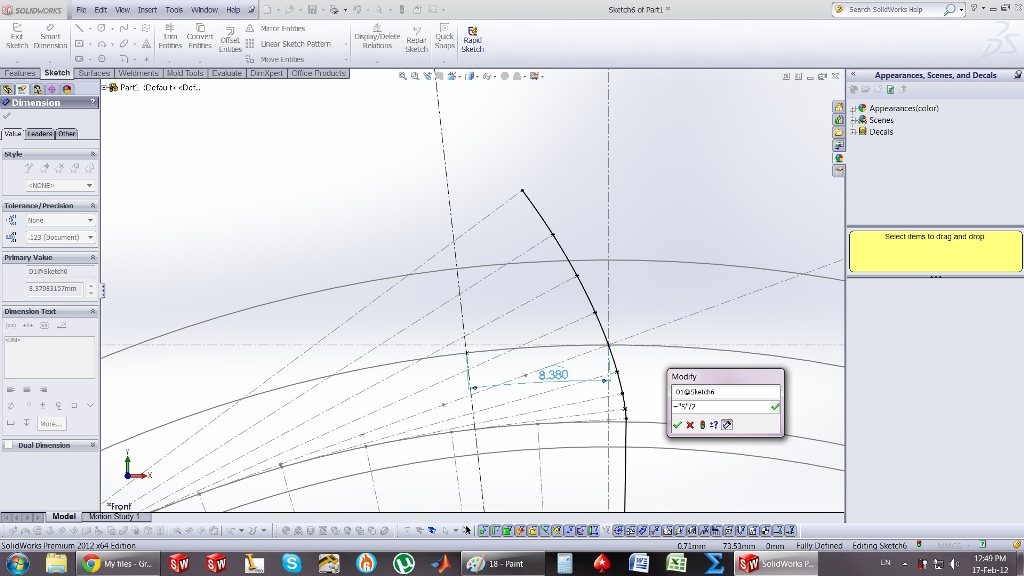19. ### Step 19:

mirror...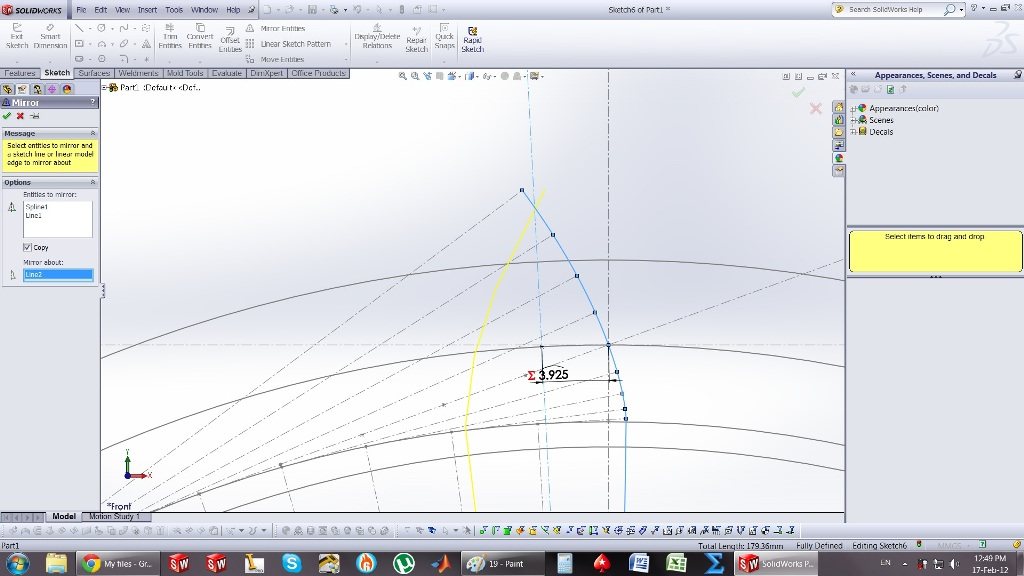20. ### Step 20:

convent entities for the 2 circles..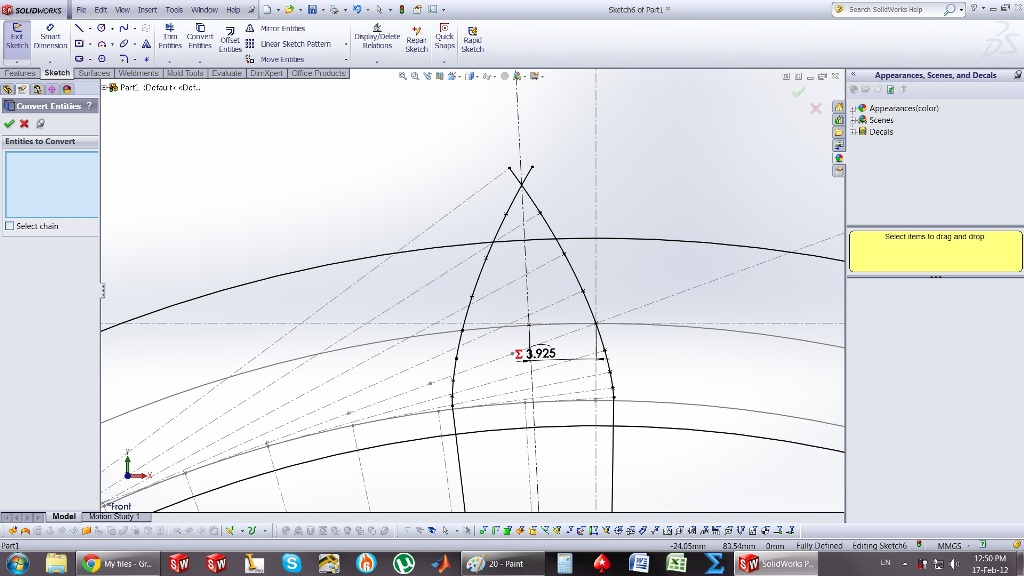21. ### Step 21:

trim...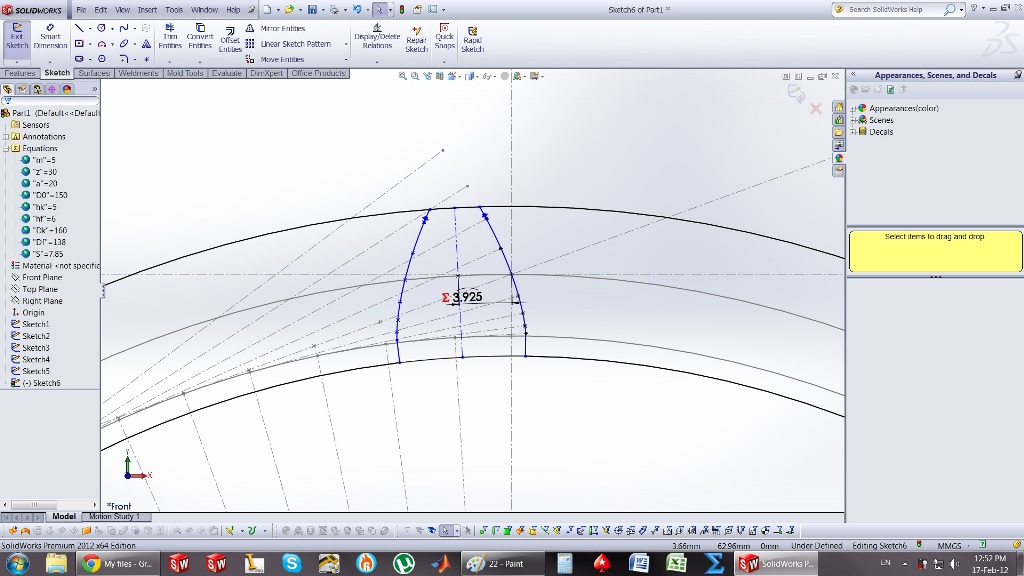22. ### Step 22:

extrude the circle...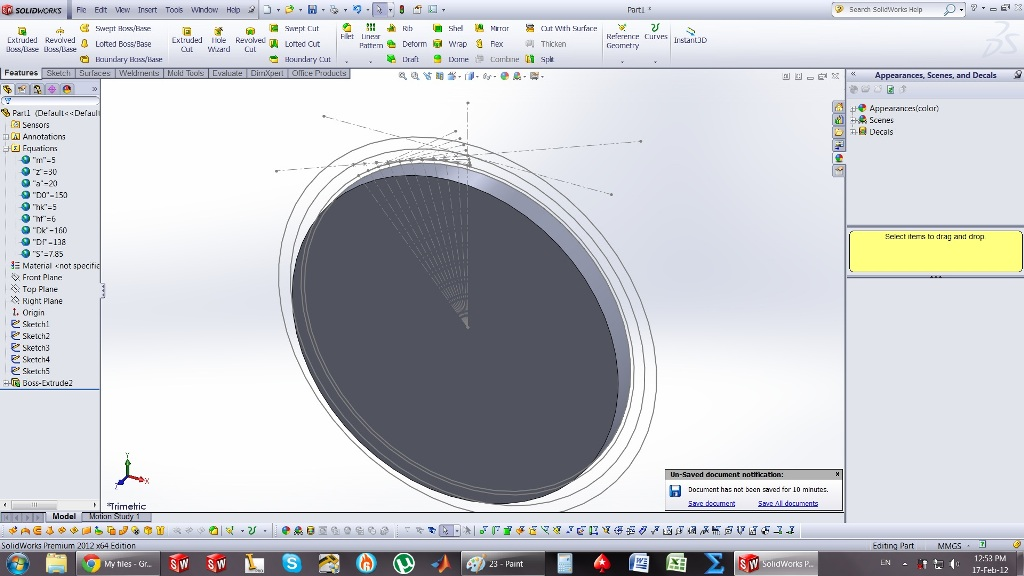23. ### Step 23:

then the tooth..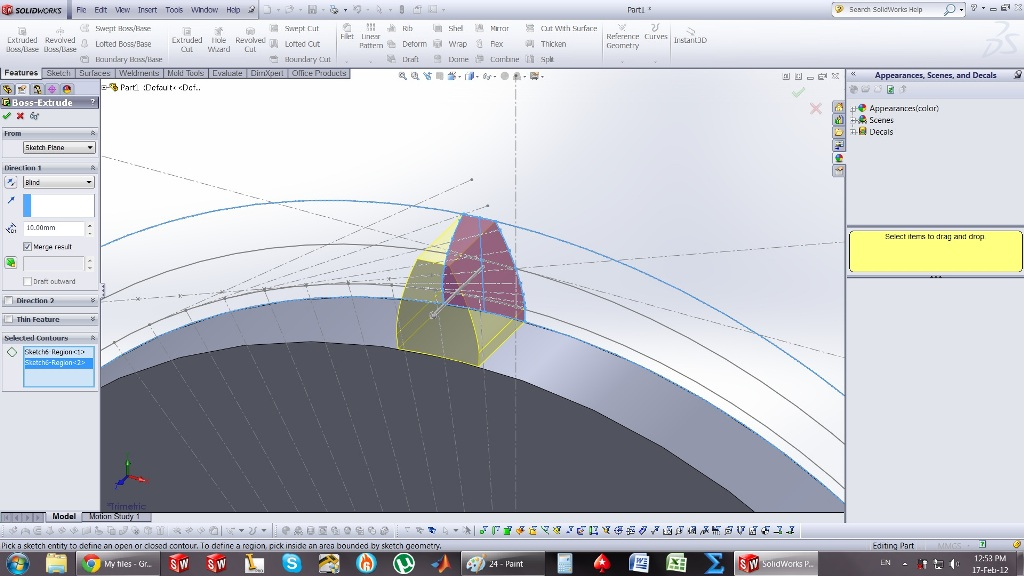24. ### Step 24: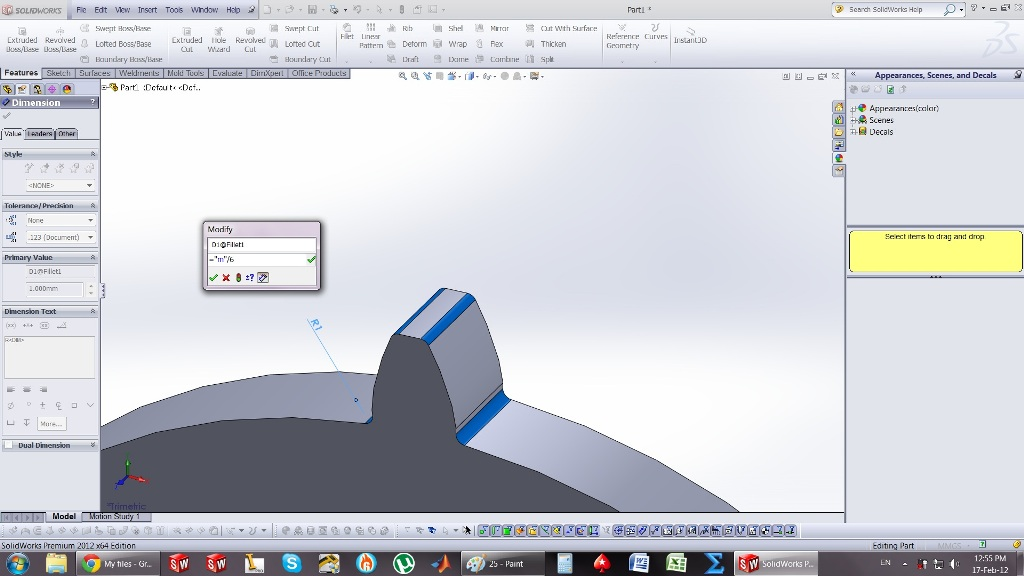25. ### Step 25: